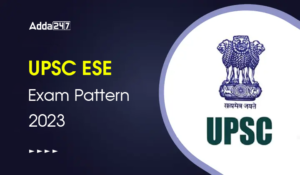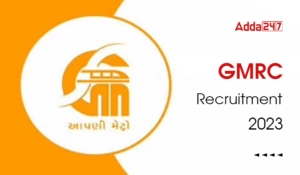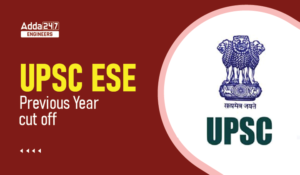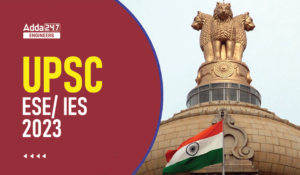Engineering Jobs   »   Civil Engineering quizs   »   UPSSSC JE QUIZ

# UPSSSC-JE’21 CE: Daily Practice Quiz. 13-October-2021

Know your strengths and practice your concepts with this quiz on UPSSSC-JE Recruitment 2021. This quiz for UPSSSC-JE Recruitment 2021 is designed specially according to UPSSSC-JE Syllabus 2021.

Quiz: Civil Engineering
Exam: UPSSSC-JE
Topic: MISCELLANEOUS

Each question carries 1 mark
Negative marking: 1/4 mark
Time: 8 Minutes

Q1. In calculating area to be deducted for bolts of 36mm diameter, the diameter of the hole shall be taken as
(a) 38.5mm
(b) 36.0mm
(c) 37.5 mm
(d) 39.0 mm

Q2. Fillet weld is not recommended if the angle between fusion face is
A. less than 60°
B. greater than 145°
C. less than 45°
D. Greater than 120°
The correct statement are :
A & D
A & B
C & B
C & D

Q3. In case of a simple supported rectangular beam of span L and loaded with a central load W, the length of elasto-plastic zone of the plastic hinge is
(a) L/5
(b) L/4
(c) L/3
(d) L/2

Q4. If L_f is load factor S is shape factor and F is factor of safety in elastic design, the following:
(a) L_f=S×F
(b) L_f=S+F
(c) L_f=S-F
(d) L_f=F-S

Q5. For the fillet weld of size ‘S’ shown in the adjoining figure the effective throat thickness is(a) 0.61 S
(b) 0.63 S
(c) 0.65 S
(d) 0.75 S

Q6. If the maximum bending moment of a simply supported slab is M kg – cm, the effective depth of the slab is
(a) M/100Q
(b) M/(10√Q)
(c) √(M/Q)
(d) √(M/(100 Q))

SOLUTION

S1. Ans.(d)
Sol. diameter of the hole or gross diameter of bolts is increased by certain allowances than nominal diameter of bolt As given below-

In case of bolt →
Nominal diameter (ϕ) Gross diameter of hole

 Nominal diameter (ϕ) Gross diameter of hole ϕ ≤ 12 mm. (ϕ) + 1 mm. 12 mm < ϕ ≤ 24 mm. (ϕ) + 2 mm. ϕ > 24 mm. (ϕ) + 3 mm.

Given, Nominal dia. of bolt (ϕ) = 36mm.
Here, ϕ>24 mm.,Hence
Gross diameter of hole = (ϕ) + 3 mm.
= 36 + 3
= 39 mm.

S2. Ans.(a)
Sol. fillet weld is not recommended if the angle between fusion faces less than 60° or more than 120°

S3. Ans.(c)
Sol. the length of elasto-plastic zone (L_P ) of the plastic hinge for simple supported beam carrying concentrated load at mid span is given by –
▭(l_P=L[ 1-1/(shape factor )] )

l_P = length of elasto-plastic zone
L = Span
Shape factor = 1.5 (for rectangular section)
l_P=L(1-1/1.5)
=L(1-10/15)
=L (1/3)
▭(l_P=L/3)

S4. Ans.(a)
Sol. Load factor = Shape factor × factor of safety
▭(L_F=S×F)
▭(Shape factor (S)=Z_P/Z_e )
Where Z_P = plastic section modulus
Z_e = Elastic section modulus

S5. Ans.(c)
Sol. ▭(effective throat thickness (t)=K.S)
S = size of weld
‘k’ depends upon angle between fusion
Here, angle of fusion given = 99°

 Angle of fusion K 60°- 90° 0.70 91° – 100° 0.65 101° – 106° 0.60 107° – 113° 0.55 114° – 120° 0.50

Hence ▭(t=0.65 S)
→ effective throat thickness should not be less than 3 mm.

S6. Ans.(d)
Sol. moment of resistance (M) = Qd² (N-mm)
M/(10×10) (kg-cm)=Qd^2
▭(d=√(M/100Q))

Sharing is caring!

Thank You, Your details have been submitted we will get back to you.
•GATE 2023 Admit Card Out, Direct Link fo...
•UPSC ESE Admit Card 2023, Download Preli...
•BHEL Engineer Trainee Syllabus and Exam ...
•UPSC ESE Exam Pattern 2023, Check UPSC E...
•GMRC Recruitment 2023, Apply Online For ...
•UPSC ESE Previous Year Cutoff, Check Yea...
•UPSC ESE/IES 2023 Notification, Apply On...